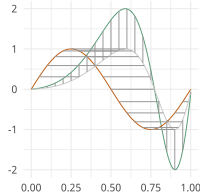# Functional Principal Least Squares¶

Partial Least Squares using SVD

moduleauthor:: J. Derek Tucker <jdtuck@sandia.gov>

fPLS.pls_svd(time, qf, qg, no, alpha=0.0)[source]

This function computes the partial least squares using SVD

Parameters: time – vector describing time samples qf – numpy ndarray of shape (M,N) of N functions with M samples qg – numpy ndarray of shape (M,N) of N functions with M samples no – number of components alpha – amount of smoothing (Default = 0.0 i.e., none) numpy ndarray f weight function g weight function smoothing value singular values